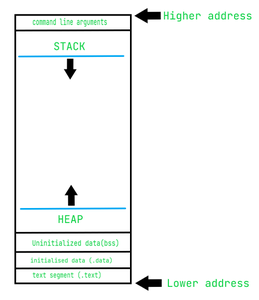Open In App

# How variable length argument works?

Variadic Function: A variadic function are the templates that take a variable-length argument. A variable-length argument is a feature that allows a function to receive any number of arguments. There are situations where a function handles a variable number of arguments according to requirements, such as:

• Sum of given numbers.
• Minimum of given numbers and many more.

A variable number of arguments are represented by three dotes (…).

Program 1:

## C

 `// C program to demonstrate the use of``// variable number of arguments``#include ``#include `` ` `// Function to find the minimum of integer``// numbers passed, ftrst argument is count``// of numbers``int` `min(``int` `arg_count, ...)``{``    ``int` `i;``    ``int` `min, a;`` ` `    ``// va_list is a type that holds the``    ``// information about variable arguments``    ``va_list` `ap;`` ` `    ``// va_start must be called before``    ``// accessing variable argument list``    ``va_start``(ap, arg_count);`` ` `    ``// Now arguments can be accessed one``    ``// by one using va_arg macro.`` ` `    ``// Initialize min as the first``    ``// argument in list``    ``min = ``va_arg``(ap, ``int``);`` ` `    ``// Traverse the rest of arguments``    ``// to find out minimum``    ``for` `(i = 2; i <= arg_count; i++)``        ``if` `((a = ``va_arg``(ap, ``int``)) < min)``            ``min = a;`` ` `    ``// va_end should be executed before``    ``// the function returns whenever``    ``// va_start has been previously``    ``// used in that function``    ``va_end``(ap);`` ` `    ``return` `min;``}`` ` `// Driver Code``int` `main()``{``    ``int` `count = 5;``    ``printf``(``"Minimum value is %d"``,``           ``min(count, 12, 67, 6, 7, 100));`` ` `    ``return` `0;``}`

Output

`Minimum value is 6`

Calling Convention: Calling convention refers to how a function is called, how parameters are passed, and how the stack is cleaned. C/C++ has a variety of calling conventions but we are only concerned with __cdecl and __stdcall.

Both are very similar but have some differences.

• __cdecl is the default calling convention of C/C++.
• __stdcall is the default calling convention Windows API functions.

Now both calling conventions pass parameters right to left. C/C++ developers choose right to left instead of left to right.

Memory Layout: The memory layout is discussed below:The only thing that needs attention is the stack and heap segment growing in the opposite direction.

1. The heap grows towards a higher address.
2. The stack grows towards the lower address. It means higher on the stack is lower in the address. When we push something on the stack, it gets the lowest immediate address in the stack.

Let’s understand this by function:

Inside main() we have a function func(arg1, arg2, arg3)
When func is called then main() is called “caller” and func() is called “callee”
Lets see its stack
caller local variable   -> lower on stack higher on address
—- (other stuffs)
arg3 (rightmost)
arg2
arg1
—-

callee local variables  -> higher on stack lower on address ^ new stack are created here not at top

In the above section, you can clearly see that the first argument gets the lowest address. This is the reason why developers chose right to left instead of left to right because the left to right calling convention will give the first argument the highest address which can cause a problem.

The first argument gets the lowest address and all parameters will have a continuous address in the stack.

Negative Subscript: [] is the subscript operator. Below are some of the important points to note about the subscript operator:

• If the subscript operator is operating on a pointer, then its behavior is different. i.e., ptr[x] means *(ptr + x) i.e., value at x*sizeof(data_type_of_pointer) ahead of ptr.
• Similarly, ptr[-x] means *(ptr – x) i.e., value at x*sizeof(data_type_of_pointer) behind ptr.

Below is an example to show how <stdarg.h> works:

## C++

 `// C++ program to implement stdarg.h``#include ``#include ``using` `namespace` `std;`` ` `// Function to find the sum of numbers``int` `sum(``int` `num, ...)``{``    ``int` `res = 0;``    ``va_list` `ap;``    ``va_start``(ap, num);``    ``for` `(``int` `i = 0; i < num; i++) {``        ``res += ``va_arg``(ap, ``int``);``    ``}`` ` `    ``// Return the resultant sum``    ``return` `res;``}`` ` `// Driver Code``int` `main()``{``    ``// First argument is the number``    ``// of arguments``    ``cout << sum(4, 6, 89, 34, 26);`` ` `    ``return` `0;``}`

Output

`155`

Explanation:
Built-in implementation has some restrictions, let’s have a look at them and see how it can be overcome. One of them is there is a need to pass the first argument mandatory, and let’s see why it is mandatory and how to avoid it.

<stdarg.h> uses va_list, va_start, va_arg and va_end. The problem can be broken into 2 parts:

• What do they do?
• How can we replicate it?

va_list: It is a typedef of char* but this is quite different, as when it is used as a type of C-string we get unpredictable results. This is because it is not common typedef. It is built-in defined.

```// arg.h
typedef char* va_list;```

va_start: This is a macro and what it does is to initialize ap, which is actually va_list type (char*) with an address ahead of the first parameter, which is arg1. This is the reason why there is a need to pass the first argument mandatory. It can be any value of any data type but for simplicity, often the number of arguments are passed. It is used to identify the address on the stack where arguments are continuous.

va_arg: This macro is quite complicated. It does two things.

• Returns the required parameter